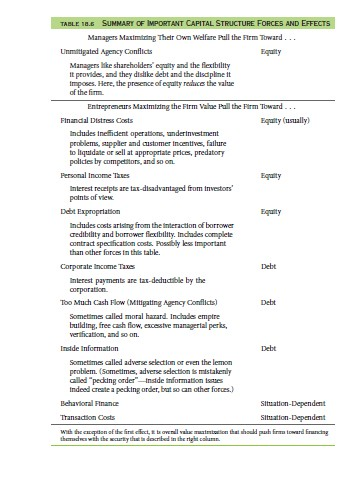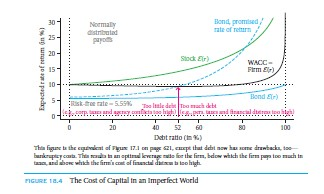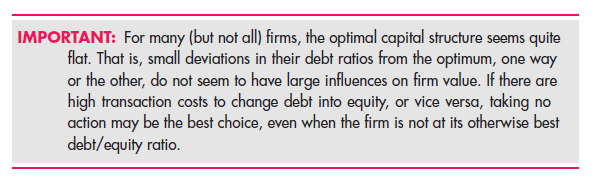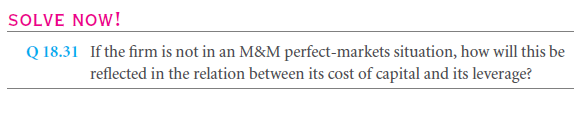By now in this chapter, we discussed the effects of various forces which are countervailing towards the value of the firm and how it affects the optimal financing of debt and equities. Let’s discuss the influence of these forces on the effective WACC of the firm. Talking about the relationship between firm value and capital cost, they are inversely related. Higher cost of capital will lead to lower value of the firm and vice versa.

Consider the value of the firm by a formula that includes the expected cash flow of the future divided by capital cost plus one. So if we keep the expected future cash flow as a constant variable, when the value of the firm will increase it will decrease the cost of capital in the firm.Talking about the role of cost of capital in debt ratio, we have already seen the same in two worlds, out of which one is perfect and the other one includes income taxes of corporate. Figure 18.4 is a perfect illustration which reflects the balance of capital structures on forces under the presence of various imperfections on the capital market.

We can notice that cost of debt and equity capital is seemingly affected by other forces which are influenced on the same. The same can be reflected by the graph as well where WACC function is said to be minimum at 52% of the debt ratio. Since the peak is flat, we can say that firm will not lead to any big mistake if the ratio is exceeded by even 10%.

But one cannot guarantee the same situation every time. When the graph of capital cost is more curved under WACC function, the introduction of sub optimal capital structure becomes really crucial and important. And therefore, it is important to relate to the order of effects that might affect the capital cost and the value of the firm in a serious notion.Links of Next Financial Accounting Topics:-### Customer Reviews

My Homework Help
Rated 5.0 out of 5 based on 510 customer reviews at